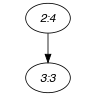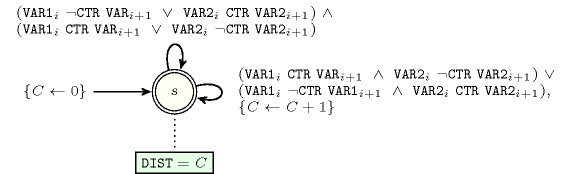## 5.131. distance_change

Origin

Derived from $\mathrm{𝚌𝚑𝚊𝚗𝚐𝚎}$.

Constraint

$\mathrm{𝚍𝚒𝚜𝚝𝚊𝚗𝚌𝚎}_\mathrm{𝚌𝚑𝚊𝚗𝚐𝚎}\left(\mathrm{𝙳𝙸𝚂𝚃},\mathrm{𝚅𝙰𝚁𝙸𝙰𝙱𝙻𝙴𝚂}\mathtt{1},\mathrm{𝚅𝙰𝚁𝙸𝙰𝙱𝙻𝙴𝚂}\mathtt{2},\mathrm{𝙲𝚃𝚁}\right)$

Synonym

$\mathrm{𝚍𝚒𝚜𝚝𝚊𝚗𝚌𝚎}$.

Arguments
 $\mathrm{𝙳𝙸𝚂𝚃}$ $\mathrm{𝚍𝚟𝚊𝚛}$ $\mathrm{𝚅𝙰𝚁𝙸𝙰𝙱𝙻𝙴𝚂}\mathtt{1}$ $\mathrm{𝚌𝚘𝚕𝚕𝚎𝚌𝚝𝚒𝚘𝚗}\left(\mathrm{𝚟𝚊𝚛}-\mathrm{𝚍𝚟𝚊𝚛}\right)$ $\mathrm{𝚅𝙰𝚁𝙸𝙰𝙱𝙻𝙴𝚂}\mathtt{2}$ $\mathrm{𝚌𝚘𝚕𝚕𝚎𝚌𝚝𝚒𝚘𝚗}\left(\mathrm{𝚟𝚊𝚛}-\mathrm{𝚍𝚟𝚊𝚛}\right)$ $\mathrm{𝙲𝚃𝚁}$ $\mathrm{𝚊𝚝𝚘𝚖}$
Restrictions
 $\mathrm{𝙳𝙸𝚂𝚃}\ge 0$ $\mathrm{𝙳𝙸𝚂𝚃}<|\mathrm{𝚅𝙰𝚁𝙸𝙰𝙱𝙻𝙴𝚂}\mathtt{1}|$ $\mathrm{𝚛𝚎𝚚𝚞𝚒𝚛𝚎𝚍}$$\left(\mathrm{𝚅𝙰𝚁𝙸𝙰𝙱𝙻𝙴𝚂}\mathtt{1},\mathrm{𝚟𝚊𝚛}\right)$ $\mathrm{𝚛𝚎𝚚𝚞𝚒𝚛𝚎𝚍}$$\left(\mathrm{𝚅𝙰𝚁𝙸𝙰𝙱𝙻𝙴𝚂}\mathtt{2},\mathrm{𝚟𝚊𝚛}\right)$ $|\mathrm{𝚅𝙰𝚁𝙸𝙰𝙱𝙻𝙴𝚂}\mathtt{1}|=|\mathrm{𝚅𝙰𝚁𝙸𝙰𝙱𝙻𝙴𝚂}\mathtt{2}|$ $\mathrm{𝙲𝚃𝚁}\in \left[=,\ne ,<,\ge ,>,\le \right]$
Purpose

$\mathrm{𝙳𝙸𝚂𝚃}$ is equal to the number of times one of the following two conditions is true $\left(1\le i:

• $\mathrm{𝚅𝙰𝚁𝙸𝙰𝙱𝙻𝙴𝚂}\mathtt{1}\left[i\right].\mathrm{𝚟𝚊𝚛}\mathrm{𝙲𝚃𝚁}\mathrm{𝚅𝙰𝚁𝙸𝙰𝙱𝙻𝙴𝚂}\mathtt{1}\left[i+1\right].\mathrm{𝚟𝚊𝚛}$ holds and $\mathrm{𝚅𝙰𝚁𝙸𝙰𝙱𝙻𝙴𝚂}\mathtt{2}\left[i\right].\mathrm{𝚟𝚊𝚛}\mathrm{𝙲𝚃𝚁}\mathrm{𝚅𝙰𝚁𝙸𝙰𝙱𝙻𝙴𝚂}\mathtt{2}\left[i+1\right].\mathrm{𝚟𝚊𝚛}$ does not hold,

• $\mathrm{𝚅𝙰𝚁𝙸𝙰𝙱𝙻𝙴𝚂}\mathtt{2}\left[i\right].\mathrm{𝚟𝚊𝚛}\mathrm{𝙲𝚃𝚁}\mathrm{𝚅𝙰𝚁𝙸𝙰𝙱𝙻𝙴𝚂}\mathtt{2}\left[i+1\right].\mathrm{𝚟𝚊𝚛}$ holds and $\mathrm{𝚅𝙰𝚁𝙸𝙰𝙱𝙻𝙴𝚂}\mathtt{1}\left[i\right].\mathrm{𝚟𝚊𝚛}\mathrm{𝙲𝚃𝚁}\mathrm{𝚅𝙰𝚁𝙸𝙰𝙱𝙻𝙴𝚂}\mathtt{1}\left[i+1\right].\mathrm{𝚟𝚊𝚛}$ does not hold.

Example
$\left(1,〈3,3,1,2,2〉,〈4,4,3,3,3〉,\ne \right)$

The $\mathrm{𝚍𝚒𝚜𝚝𝚊𝚗𝚌𝚎}_\mathrm{𝚌𝚑𝚊𝚗𝚐𝚎}$ constraint holds since the following condition ($\mathrm{𝙳𝙸𝚂𝚃}=1$) is verified: $\left\{\begin{array}{c}\mathrm{𝚅𝙰𝚁𝙸𝙰𝙱𝙻𝙴𝚂}\mathtt{1}\left[3\right].\mathrm{𝚟𝚊𝚛}=1\ne \mathrm{𝚅𝙰𝚁𝙸𝙰𝙱𝙻𝙴𝚂}\mathtt{1}\left[4\right].\mathrm{𝚟𝚊𝚛}=2\wedge \hfill \\ \mathrm{𝚅𝙰𝚁𝙸𝙰𝙱𝙻𝙴𝚂}\mathtt{2}\left[3\right].\mathrm{𝚟𝚊𝚛}=3=\mathrm{𝚅𝙰𝚁𝙸𝙰𝙱𝙻𝙴𝚂}\mathtt{1}\left[4\right].\mathrm{𝚟𝚊𝚛}=3\hfill \end{array}\right\$.

Typical
 $\mathrm{𝙳𝙸𝚂𝚃}>0$ $|\mathrm{𝚅𝙰𝚁𝙸𝙰𝙱𝙻𝙴𝚂}\mathtt{1}|>1$ $\mathrm{𝙲𝚃𝚁}\in \left[=,\ne \right]$
Symmetries
• Arguments are permutable w.r.t. permutation $\left(\mathrm{𝙳𝙸𝚂𝚃}\right)$ $\left(\mathrm{𝚅𝙰𝚁𝙸𝙰𝙱𝙻𝙴𝚂}\mathtt{1},\mathrm{𝚅𝙰𝚁𝙸𝙰𝙱𝙻𝙴𝚂}\mathtt{2}\right)$ $\left(\mathrm{𝙲𝚃𝚁}\right)$.

• One and the same constant can be added to the $\mathrm{𝚟𝚊𝚛}$ attribute of all items of $\mathrm{𝚅𝙰𝚁𝙸𝙰𝙱𝙻𝙴𝚂}\mathtt{1}$.

• One and the same constant can be added to the $\mathrm{𝚟𝚊𝚛}$ attribute of all items of $\mathrm{𝚅𝙰𝚁𝙸𝙰𝙱𝙻𝙴𝚂}\mathtt{2}$.

Arg. properties

Functional dependency: $\mathrm{𝙳𝙸𝚂𝚃}$ determined by $\mathrm{𝚅𝙰𝚁𝙸𝙰𝙱𝙻𝙴𝚂}\mathtt{1}$, $\mathrm{𝚅𝙰𝚁𝙸𝙰𝙱𝙻𝙴𝚂}\mathtt{2}$ and $\mathrm{𝙲𝚃𝚁}$.

Usage

Measure the distance between two sequences according to the $\mathrm{𝚌𝚑𝚊𝚗𝚐𝚎}$ constraint.

Remark

We measure that distance with respect to a given constraint and not according to the fact that the variables are assigned distinct values.

Keywords
Arc input(s)

$\mathrm{𝚅𝙰𝚁𝙸𝙰𝙱𝙻𝙴𝚂}\mathtt{1}$/ $\mathrm{𝚅𝙰𝚁𝙸𝙰𝙱𝙻𝙴𝚂}\mathtt{2}$

Arc generator
$\mathrm{𝑃𝐴𝑇𝐻}$$↦\mathrm{𝚌𝚘𝚕𝚕𝚎𝚌𝚝𝚒𝚘𝚗}\left(\mathrm{𝚟𝚊𝚛𝚒𝚊𝚋𝚕𝚎𝚜}\mathtt{1},\mathrm{𝚟𝚊𝚛𝚒𝚊𝚋𝚕𝚎𝚜}\mathtt{2}\right)$

Arc arity
Arc constraint(s)
$\mathrm{𝚟𝚊𝚛𝚒𝚊𝚋𝚕𝚎𝚜}\mathtt{1}.\mathrm{𝚟𝚊𝚛}\mathrm{𝙲𝚃𝚁}\mathrm{𝚟𝚊𝚛𝚒𝚊𝚋𝚕𝚎𝚜}\mathtt{2}.\mathrm{𝚟𝚊𝚛}$
Graph property(ies)
$\mathrm{𝐃𝐈𝐒𝐓𝐀𝐍𝐂𝐄}$$=\mathrm{𝙳𝙸𝚂𝚃}$

Graph model

Within the Arc input(s) slot, the character / indicates that we generate two distinct graphs. The graph property $\mathrm{𝙳𝙸𝚂𝚃𝙰𝙽𝙲𝙴}$ measures the distance between two digraphs ${G}_{1}$ and ${G}_{2}$. This distance is defined as the sum of the following quantities:

• The number of arcs of ${G}_{1}$ that do not belong to ${G}_{2}$,

• The number of arcs of ${G}_{2}$ that do not belong to ${G}_{1}$.

Part (A) of Figure 5.131.1 gives the final graph associated with the sequence $\mathrm{𝚟𝚊𝚛}$-3,$\mathrm{𝚟𝚊𝚛}$-3,$\mathrm{𝚟𝚊𝚛}$-1,$\mathrm{𝚟𝚊𝚛}$-2,$\mathrm{𝚟𝚊𝚛}$-2 (i.e., the second argument of the constraint of the Example slot), while part (B) shows the final graph corresponding to $\mathrm{𝚟𝚊𝚛}$-4,$\mathrm{𝚟𝚊𝚛}$-4,$\mathrm{𝚟𝚊𝚛}$-3,$\mathrm{𝚟𝚊𝚛}$-3,$\mathrm{𝚟𝚊𝚛}$-3 (i.e., the third argument of the constraint of the Example slot). Since arc $3\to 4$ belongs to the first final graph but not to the second one, the distance between the two final graphs is equal to 1.

##### Figure 5.131.1. Final graphs of the $\mathrm{𝚍𝚒𝚜𝚝𝚊𝚗𝚌𝚎}_\mathrm{𝚌𝚑𝚊𝚗𝚐𝚎}$ constraint(a) (b)
Automaton

Figure 5.131.2 depicts the automaton associated with the $\mathrm{𝚍𝚒𝚜𝚝𝚊𝚗𝚌𝚎}_\mathrm{𝚌𝚑𝚊𝚗𝚐𝚎}$ constraint. Let $\left(\mathrm{𝚅𝙰𝚁}{\mathtt{1}}_{i},\mathrm{𝚅𝙰𝚁}{\mathtt{1}}_{i+1}\right)$ and $\left(\mathrm{𝚅𝙰𝚁}{\mathtt{2}}_{i},\mathrm{𝚅𝙰𝚁}{\mathtt{2}}_{i+1}\right)$ respectively be the ${i}^{th}$ pairs of consecutive variables of the collections $\mathrm{𝚅𝙰𝚁𝙸𝙰𝙱𝙻𝙴𝚂}\mathtt{1}$ and $\mathrm{𝚅𝙰𝚁𝙸𝙰𝙱𝙻𝙴𝚂}\mathtt{2}$. To each quadruple $\left(\mathrm{𝚅𝙰𝚁}{\mathtt{1}}_{i},\mathrm{𝚅𝙰𝚁}{\mathtt{1}}_{i+1},\mathrm{𝚅𝙰𝚁}{\mathtt{2}}_{i},\mathrm{𝚅𝙰𝚁}{\mathtt{2}}_{i+1}\right)$ corresponds a 0-1 signature variable ${S}_{i}$. The following signature constraint links these variables:

$\left(\left(\mathrm{𝚅𝙰𝚁}{\mathtt{1}}_{i}=\mathrm{𝚅𝙰𝚁}{\mathtt{1}}_{i+1}\right)\wedge \left(\mathrm{𝚅𝙰𝚁}{\mathtt{2}}_{i}\ne \mathrm{𝚅𝙰𝚁}{\mathtt{2}}_{i+1}\right)\right)\vee$

$\left(\left(\mathrm{𝚅𝙰𝚁}{\mathtt{1}}_{i}\ne \mathrm{𝚅𝙰𝚁}{\mathtt{1}}_{i+1}\right)\wedge \left(\mathrm{𝚅𝙰𝚁}{\mathtt{2}}_{i}=\mathrm{𝚅𝙰𝚁}{\mathtt{2}}_{i+1}\right)\right)⇔{S}_{i}$.

##### Figure 5.131.2. Automaton of the $\mathrm{𝚍𝚒𝚜𝚝𝚊𝚗𝚌𝚎}_\mathrm{𝚌𝚑𝚊𝚗𝚐𝚎}$ constraint##### Figure 5.131.3. Hypergraph of the reformulation corresponding to the automaton of the $\mathrm{𝚍𝚒𝚜𝚝𝚊𝚗𝚌𝚎}_\mathrm{𝚌𝚑𝚊𝚗𝚐𝚎}$ constraint